# Other Types of Cipher

### Principle¶

The Bacon password uses two different fonts, representing A and B, combined with an encryption table for encryption and decryption.

a AAAAA g AABBA n ABBAA t BAABA

| b AAAAB | h. | AABBB | o | ABBAB | uv | BAABB | | c | AAABA | ij | ABAAA | p | ABBBA | w | BABAA | | d | AAABB | k | ABAAB | q | ABBBB | x | BABAB | | e | AABAA | l | ABABA | r | BAAAA | y | BABBA | | f AABAB m ABABB | s BAAAB z BABBB

The above is a commonly used encryption table. There is another type of encryption table that can be thought of as sorting 26 letters from 0 to 25, expressed in binary, with A representing 0 and B representing 1.

The following paragraph is the encrypted content of plain text steganography, the normal font is A, and the bold is B:

To encode a message each letter of the plaintext is replaced by a group of five of the letters 'A' or 'B'.

It can be seen that the Bacon password mainly has the following characteristics.

• only two characters
• the length of each segment is 5
• Encrypted content will have a special font, or case.

### Principle¶

The fence password divides the plaintext to be encrypted into N groups, and then connects the first word of each group to form an irregular word. Here is an example

明文：THERE IS A CIPHER


Changed after removing the space

THEREISACIPHER


Divided into two columns, two groups get

TH IS EI SA CI PH ER


First take the first letter and then take the second letter

TEESCPE

HRIAIHR


Connected together

TEESCPEHRIAIHR


The above plain text can also be divided into 2 columns.

THEREIS ACIPHER


Combine to get ciphertext

TAHCEIRPEHIESR


## 曲路密码¶

### Principle¶

Curve Cipher is a transposition password that requires the two parties to agree on the key (that is, the path of the curve). An example is given below

明文：The quick brown fox jumps over the lazy dog


Fill in 5 rows and 7 lists (pre-agreed the number of rows and columns filled)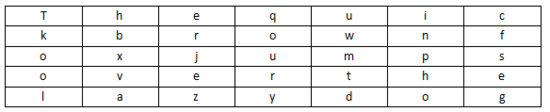Encrypted loop line (pre-agreed the number of rows and columns filled)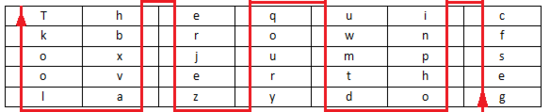密 文: gesfc inpho dtmwu qoury zejre hbxva lookT


## Column Shift Encryption¶

### Principle¶

Column Transposition Cipher is a relatively simple and easy-to-implement transposition cipher that mixes plaintext into ciphertext through a simple rule. An example is given below.

We use the clear text The quick brown fox jumps over the lazy dog, the key how are u as an example:

Fill in the clear line with 5 rows and 7 lists (pre-agreed the number of rows and columns to be filled in. If the plain text cannot be filled in, the form can be filled with a letter)Key: how are u, press how are u to number in the order in which the alphabet appears. We have a for 1, e for 2, h for 3, o for 4, and r for 5. u is 6, and w is 7, so write a column first, followed by column e, and so on. The result is cipher text: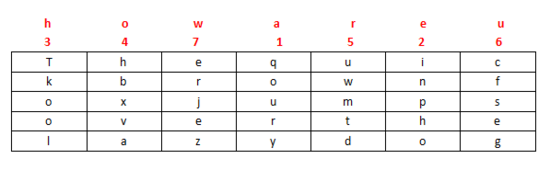### Principle¶

This password, also known as cloud shadow password, uses 0, 1, 2, 4, 8 four digits, where 0 is used to indicate the interval, and other numbers can be expressed by addition: 28=10,124=7,18=9 Then use 1->26 to indicate A->Z.

It can be seen that the password has the following characteristics

• only 0, 1, 2, 4, 8

### Examples¶

Here we take CFF 2016 shadow password as an example, the topic

8842101220480224404014224202480122

We divide by 0, as follows

Content Numbers Characters

| 88421 | 8 + 8 + 4 + 2 + 1 = 23 W | | 122 | 1 + 2 + 2 = 5 | E | | 48 | 4 + 8 = 12 | L | | 2244 | 2 + 2 + 4 + 4 = 12 | L | | 4 | 4 | D |

| 142242 | 1 + 4 + 2 + 2 + 4 + 2 = 15 | O | | 248 | 2+4+8=14 | N |

| 122 | 1 + 2 + 2 = 5 | E |

So the final flag is WELLDONE.

## JSFuck¶

### Principle¶

JSFuck can write JavaScript programs with only 6 characters []()!+. For example, we want to use JSFuck to implement the alert(1) code as follows

[][(![]+[])[+[[+[]]]]+([][[]]+[])[+[[!+[]+!+[]+!+[]+!+[]+!+[]]]]+(![]+[])[+[[!+[]+!+[]]]]+(!![]+[])[+[[+[]]]]+(!![]+[])[+[[!+[]+!+[]+!+[]]]]+(!![]+[])[+[[+!+[]]]]][([][(![]+[])[+[[+[]]]]+([][[]]+[])[+[[!+[]+!+[]+!+[]+!+[]+!+[]]]]+(![]+[])[+[[!+[]+!+[]]]]+(!![]+[])[+[[+[]]]]+(!![]+[])[+[[!+[]+!+[]+!+[]]]]+(!![]+[])[+[[+!+[]]]]]+[])[+[[!+[]+!+[]+!+[]]]]+([][(![]+[])[+[[+[]]]]+([][[]]+[])[+[[!+[]+!+[]+!+[]+!+[]+!+[]]]]+(![]+[])[+[[!+[]+!+[]]]]+(!![]+[])[+[[+[]]]]+(!![]+[])[+[[!+[]+!+[]+!+[]]]]+(!![]+[])[+[[+!+[]]]]]+[])[+[[!+[]+!+[]+!+[]+!+[]+!+[]+!+[]]]]+([][[]]+[])[+[[+!+[]]]]+(![]+[])[+[[!+[]+!+[]+!+[]]]]+(!![]+[])[+[[+[]]]]+(!![]+[])[+[[+!+[]]]]+([][[]]+[])[+[[+[]]]]+([][(![]+[])[+[[+[]]]]+([][[]]+[])[+[[!+[]+!+[]+!+[]+!+[]+!+[]]]]+(![]+[])[+[[!+[]+!+[]]]]+(!![]+[])[+[[+[]]]]+(!![]+[])[+[[!+[]+!+[]+!+[]]]]+(!![]+[])[+[[+!+[]]]]]+[])[+[[!+[]+!+[]+!+[]]]]+(!![]+[])[+[[+[]]]]+([][(![]+[])[+[[+[]]]]+([][[]]+[])[+[[!+[]+!+[]+!+[]+!+[]+!+[]]]]+(![]+[])[+[[!+[]+!+[]]]]+(!![]+[])[+[[+[]]]]+(!![]+[])[+[[!+[]+!+[]+!+[]]]]+(!![]+[])[+[[+!+[]]]]]+[])[+[[!+[]+!+[]+!+[]+!+[]+!+[]+!+[]]]]+(!![]+[])[+[[+!+[]]]]]((![]+[])[+[[+!+[]]]]+(![]+[])[+[[!+[]+!+[]]]]+(!![]+[])[+[[!+[]+!+[]+!+[]]]]+(!![]+[])[+[[+!+[]]]]+(!![]+[])[+[[+[]]]]+([][(![]+[])[+[[+[]]]]+([][[]]+[])[+[[!+[]+!+[]+!+[]+!+[]+!+[]]]]+(![]+[])[+[[!+[]+!+[]]]]+(!![]+[])[+[[+[]]]]+(!![]+[])[+[[!+[]+!+[]+!+[]]]]+(!![]+[])[+[[+!+[]]]]]+[])[+[[+!+[]]]+[[!+[]+!+[]+!+[]+!+[]+!+[]]]]+[+!+[]]+([][(![]+[])[+[[+[]]]]+([][[]]+[])[+[[!+[]+!+[]+!+[]+!+[]+!+[]]]]+(![]+[])[+[[!+[]+!+[]]]]+(!![]+[])[+[[+[]]]]+(!![]+[])[+[[!+[]+!+[]+!+[]]]]+(!![]+[])[+[[+!+[]]]]]+[])[+[[+!+[]]]+[[!+[]+!+[]+!+[]+!+[]+!+[]+!+[]]]])()


Some other basic expressions:

false       =>  ![]

true        =>  !![]

undefined   =>  [][[]]

NaN =&gt; + [! []]
0           =>  +[]

1           =>  +!+[]

2           =>  !+[]+!+[]

10          =>  [+!+[]]+[+[]]

Array       =>  []

Number      =>  +[]

String      =>  []+[]

Boolean     =>  ![]

Function    =>  []["filter"]

eval        =>  []["filter"]["constructor"]( CODE )()

window      =>  []["filter"]["constructor"]("return this")()


## BrainFuck¶

### Principle¶

Brainfuck, a minimal computer language, was created in 1993 by Urban Müller. Let's take an example if we want to print Hello World on the screen! , then the corresponding program is as follows. For the principles, you can search online for your own interest.

++++++++++[>+++++++>++++++++++>+++>+<<<<-]

>++.>+.+++++++..+++.>++.<<+++++++++++++++.

>.+++.------.--------.>+.>.


Corresponding to it is ook.

### Principle¶

The pigsty password is a simple alternative password based on the grid. The grid is as follows

Let's take an example, such as the plain text is X marks the spot, then the ciphertext is as follows

### Principle¶

This password comes from the Sherlock Holmes collection. Each dancing villain actually corresponds to one of the twenty-six letters in English, while the flag in the hand of the villain indicates that the letter is the last letter of the word, if it is just a word instead of a sentence, or a sentence The last word in the word, the last letter in the word does not have to be raised.

The so-called keyboard password is encrypted using a mobile phone keyboard or a computer keyboard.

The phone keyboard encryption method is that there are 3-4 letters on each number key, and the two-digit number is used to represent the letter. For example: ru is represented by the phone keypad: 7382, then you can know here, the phone keyboard encryption method is impossible. Start with 1, the second digit cannot exceed 4, refer to this when decrypting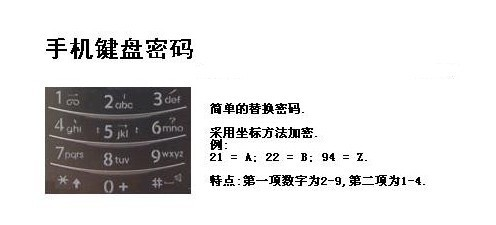Another way to encrypt your phone's keyboard is the "sound" style (this may vary depending on the phone). Refer to the phone keypad for details. For example, "Number" means: 748 94. Pressing these numbers on the phone keypad will bring out the pinyin of "number".

### Computer keyboard board¶

The computer keyboard is encrypted and uses the square of the computer's board.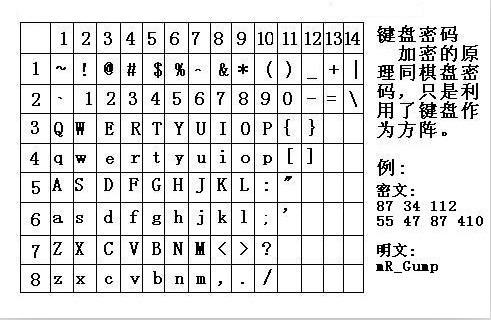### Computer keyboard coordinates¶

Encryption of the computer keyboard coordinates, using the letter lines and numbers on the keyboard to encrypt, for example: bye using the computer keyboard XY means: 351613

![Computer keyboard coordinate encryption] (./figure/computer-xy.jpg)

### Computer keyboard QWE¶

Computer keyboard QWE encryption method is to replace the order of the keyboard with the alphabet.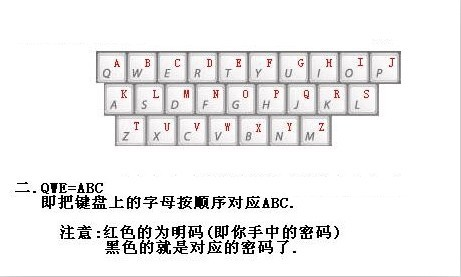### Keyboard layout encryption¶

Simply put, it is encrypted according to how the given character looks on the keyboard.

### 0CTF 2014 classic¶

> Xiaoding Ding found himself in a strange room with only a door with strange characters in front of him. He found a password lock on the side of the door, which seemed to require a password to open the door. . 4esxcft5 rdcvgt 6tfc78uhg 098ukmnb

Found so chaotic, but also including the number and letter guess may be the keyboard password, try to draw in the alphabetical order on the keyboard, you can get 0ops, guess is the flag.

### 2017 xman trials - one two three, wood people¶

> I count 123 wood people, and I will be deducted if I don't act.

23731263111628163518122316391715262121

There are obvious hints 123 in the title, then it is natural to think of the computer keyboard coordinate password in the keyboard password. You can find that the second digits of the first few digits are in the range of 1-3, which also verifies our guess. then

23-x

73-m

12-a

63-n

11-q

No, the password format is xman{, the fourth character is {, so I look at the position of {, which does not have a corresponding abscissa, but if we manually treat it as 11, then 111 is {. Then push back in turn and find that it is feasible. Finally, treat 121 as } to get the flag.

xman{hintisenough}


From here we can see that we still have to pay attention to mobility, and we cannot simply copy some existing knowledge.

### Title¶

• Experiment with strange text messages